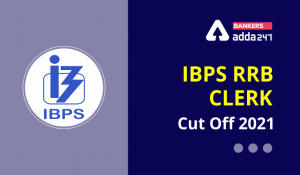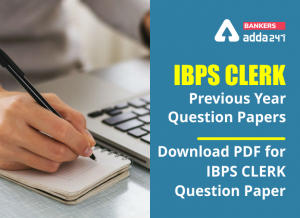Latest Banking jobs   »   How to Solve Inequality Questions in...

# How to Solve Inequality Questions in Reasoning for Bank Exams?

### What is Inequality in Reasoning?

Let us uderstand inequality with the help of an example: We know that the result of multiplication between 5 and 3 and number 15 are equal. Since they are equal it is an equality. In the same way, 5 × 5 ≠ 15. Here the product of 5 and 5 is not equal to the number 15. And since they are not equal, it is an inequality.

Also Check,

### Why it is important for bank exams?

Inequalities is one of the topics where you can get full marks very easily. It is a common topic for all competitive exams. We can expect 3 to 5 questions from Inequality in every PO and Clerk level examination.

### Questions based on the inequalities of commonly two types.

1. Direct inequalities and
2. Coded inequalities

Before discussing details for solving Inequalities questions, lets check the meaning of certain symbols in below table:–To check the different relationship, we are presenting some different statement and conclusions in below table. From given below table you will get clear concept of relationship between two letters.### Priority of Symbols in Inequality

1. > ≥ =
For ex- If A>K≥M=O
Then, A> M and T>O
2. < ≤ =
For ex- If P<X≤V=Y
Then, P<Y and P<V
3. > < (No relation)
For ex- If Q>K<L
Then there will be no relation between Q and L.
4. > ≤ (No relation)
For ex- If O>J≤H
Then there will be no relation between O and H.
5. < > (No relation)
For ex- If F<E>Q
Then there will be no relation between F and Q.
6. < ≥ (No relation)
For ex- If D<S≥Z
Then there will be no relation between D and Z.

### Either- or case

In equality it is very important condition. Mostly students make mistakes in this condition. For clear concept we are giving example of “either-or”
1st condition for “either-or” is both conclusions should be wrong.
2nd condition is that variables of both conclusions should be same.
Eg. :–
Statement: P≥Q=R
Conclusion: (a) P > R (b) P = R

In above example, relation between P and R is P≥R. But both the conclusions are wrong and both have same variables. And by combining both conclusions you will get the actual relation between A and C which comes from statement.

2. Statement: P=Q≥R≥S=T
Conclusion I: (a)P>T (b)P=T
From the above statement it is clear that P is either equals to T or P is greater than T ,So individually both the conclusions are wrong but by combining them we will get that P is either greater than or equals to T (P≥T).
Conclusion II: (a) Q>S (b) Q=S
Similarly from the above statement for conclusion II we can see that there is an either / or case between Q and S, So Q either will be greater than or equals to S.

### Complicated case of “Either-or”

Statement: H≥M≤V=K
Conclusions: (1) H<K (2) H≥K

In the above statement we cannot find the relation between H and K. There may be three possibilities of relation between H and K.
i.e. (a) H>K (b) H<K (c) H=K
And we are getting all possibilities by combining both conclusions. So, this is also one case of “Either-or”.

Statement: F<T≤N,F>S,M≤T<G
Conclusions: I.M≥S II. S>M
In the above question by combining the statements together we get S<F<T≥M. So we cannot find the relation between M and S. As there can be three possible cases: M is either greater, lesser or equals to S. In conclusions I and II we can find all the three possible cases so the answer will be either conclusion I or II follow.

Statement: L≥K<E≥A>F≥B
Conclusions: I.L<B II.B≤L
This is another example showing that no direct relation is found between B and L and all the three possible conditions as L>B, L<B or L=B can be there. So the answer will be either conclusion I or II follow.

### Example of coded Inequalities

Directions (1- 3): In the following questions, the symbols @, &, %, \$ and # are used with the following meaning as illustrated below:
‘P @ Q’ means ‘P is not smaller than Q’
‘P &Q’ means ‘P is neither greater than nor equal to Q’
‘P# Q’ means ‘P is neither greater than nor smaller than Q’
‘P \$ Q’ means ‘P is not greater than Q’
‘P % Q’ means ‘P is neither smaller than nor equal to Q’.
Now in each of the following questions assuming the given statements to be true, find which of the three conclusions follow and give answer accordingly.

Q1. Statements: R @ V, V \$ J, J &K
Conclusions I. K % R II. J @ R III. K % V
(a) Only I is true
(b) Only II is true
(c) Only I and II are true
(d) Only III is true
(e) None of these
S1.Ans.(d)

Q2. Statements: D % H, H @ V, V \$ W
Conclusions: I. H % W II. D % V III. D % W
(a) Only I is true
(b) Only II is true
(c) Only I and II are true
(d) All are true
(e) None of these
S2.Ans.(b)

Q3. Statements: M \$ T, T& J, J #N
Conclusions: I. N % M II. J % M III. M \$ N
(a) Only I is true
(b) Only II is true
(c) Only I and II are true
(d) All are true
(e) None of these
S3.Ans.(c)

Click Here to Register for Bank Exams 2020 Preparation Material

Practice with,

### FAQ on Inequality

Q1- How many questions asked in Bank prelims exams?
Ans. There can be 3-5 questions of Inequality in reasoning section of prelims examination.

Q2- Are these questions asked in the Bank PO Mains exam?
Ans. No, but sometimes 3-4 questions of coded inequality ask in clerk mains level examination.

#### Congratulations!Download Hindu Review of October 2021: Free PDFIncorrect details? Fill the form again here

Download Hindu Review of October 2021: Free PDF

Thank You, Your details have been submitted we will get back to you.
•IBPS RRB Cut Off 2022, Previous Year Cut...
•24th September Current Affairs Quiz for ...
•23rd September Daily Current Affairs 202...
•22nd September Daily Current Affairs 202...
•IBPS Clerk Previous Year Question Papers...
•IBPS PO Syllabus 2022 & Exam Pattern...